Redundant Rows: Rank of a Matrix

Same example for water and water complexes: use the matrix watmat to store molecular formulas for each type of molecule in the system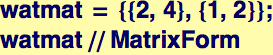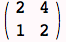The vector molvec is used to store the number of each kind of molecule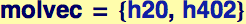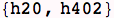The vector atomvec is used to store the number of each atomic species that is present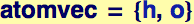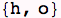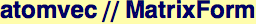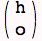The vector eq is now defined and its two elements are equations that give the number of hydrogen atoms and the number of oxygen atoms: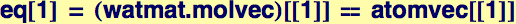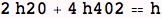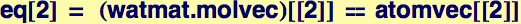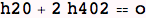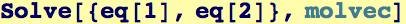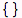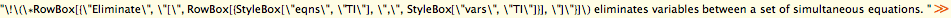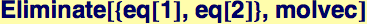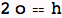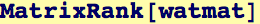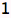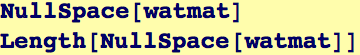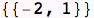Created by Wolfram Mathematica 6.0  (31 August 2007)